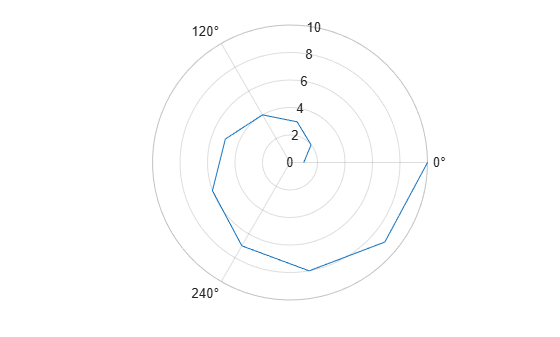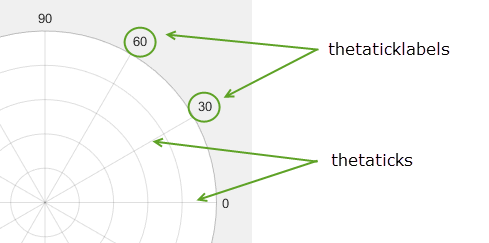# thetaticks

## 语法

``thetaticks(ticks)``
``tv = thetaticks``
``thetaticks('auto')``
``thetaticks('manual')``
``m = thetaticks('mode')``
``___ = thetaticks(pax,___)``

## 说明

``thetaticks(ticks)` 设置 theta 轴刻度值，这些值是沿 theta 轴出现刻度线和网格线的位置。指定 `ticks` 为递增值向量；例如 `[0 90 180 270]`。此命令作用于当前坐标区。`
``tv = thetaticks` 以向量形式返回当前 theta 轴刻度值。`

``thetaticks('auto')` 设置自动模式，使坐标区确定 theta 轴刻度值。如果您更改了刻度值，然后又想将它们设置回默认值，则可以使用此选项。 `
``thetaticks('manual')` 设置手动模式，将 theta 轴刻度值冻结在当前值。如果您希望在调整坐标区大小或者向坐标区添加新数据时保留当前刻度值，则可以使用此选项。`
``m = thetaticks('mode')` 返回当前 theta 轴刻度标签模式：`'auto'` 或 `'manual'`。默认情况下，模式为自动，除非您指定刻度值或将模式更改为手动。`

``___ = thetaticks(pax,___)` 使用 `pax` 指定的坐标区，而不是使用当前坐标区。指定 `pax` 作为上述任何语法的第一个输入参数。`

## 示例

```theta = linspace(0,2*pi); rho = theta/10; polarplot(theta,rho) thetaticks([0 90 180 270]) thetaticklabels({'East','North','West','South'})``````theta = linspace(0,2*pi); rho = theta/10; polarplot(theta,rho) thetaticks([0 30 60 90 180 210 240 270])``````theta = 0:0.01:2*pi; rho = sin(2*theta).*cos(2*theta); polarplot(theta,rho) thetaticks(0:15:360)``````polarplot(1:10) thetaticks([0 120 240])````thetaticks('auto')````pax = polaraxes; thetaticks(pax,0:45:360)``````theta = 0:0.01:2*pi; rho = sin(2*theta).*cos(2*theta); polarplot(theta,rho) thetaticks([])```## 输出参数

• `'auto'` - 坐标区自动确定 theta 轴刻度值。

• `'manual'` - 坐标区使用手动指定的 theta 轴刻度值。

## 详细信息

### 刻度值## 算法

`thetaticks` 函数设置并查询与 theta 轴刻度值有关的多个坐标区属性。